Function Repository Resource:

# EvenPermutations

Get even permutations of a list

Contributed by: Ed Pegg Jr
 ResourceFunction["EvenPermutations"][list] returns the even permutations of list. ResourceFunction["EvenPermutations"][list,count] returns count even permutations of list.

## Details

An even permutation is a permutation created by an even number of two-element swaps.
If the list has length 9 or less, all even permutations will be returned. If the list has length longer than 9, a random sampling of even permutations will be returned.

## Examples

### Basic Examples (2)

The even permutations of {1,2,3,4}:

 In:=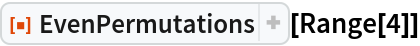Out=The even permutations of {2,1,3,4}:

 In:=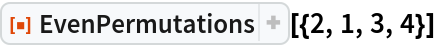Out=The even permutations of an odd permutation have signature -1:

 In:=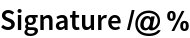Out=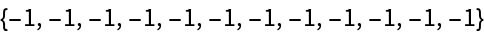Ten even permutations of {1,…,15}:

 In:=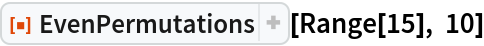Out=### Possible Issues (1)

For a list of 12 items, rather than returning 12!/2 = 239500800 items, a sampling of even permutations is given:

 In:=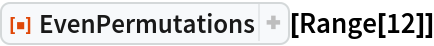Out=### Neat Examples (1)

Use even permutations to embed an icosahedron within a tetrahedron:

 In:=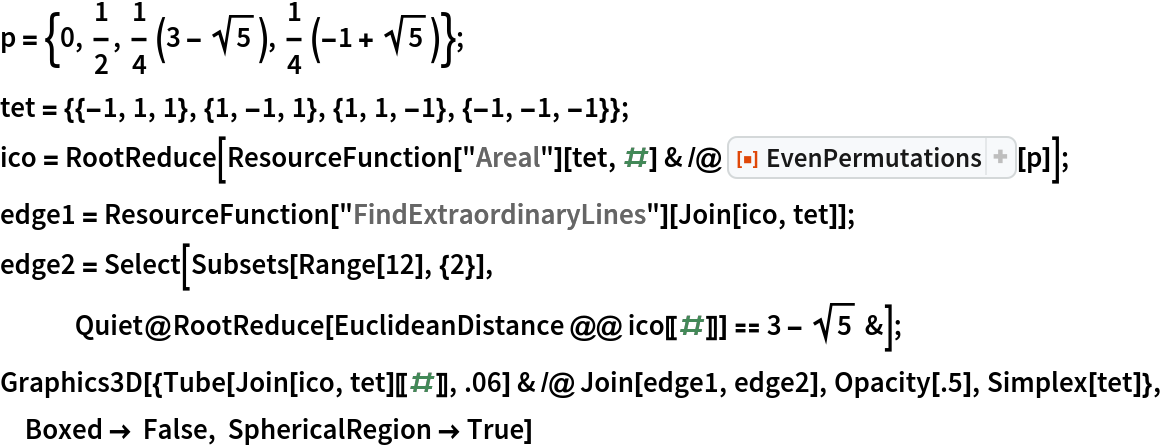Out=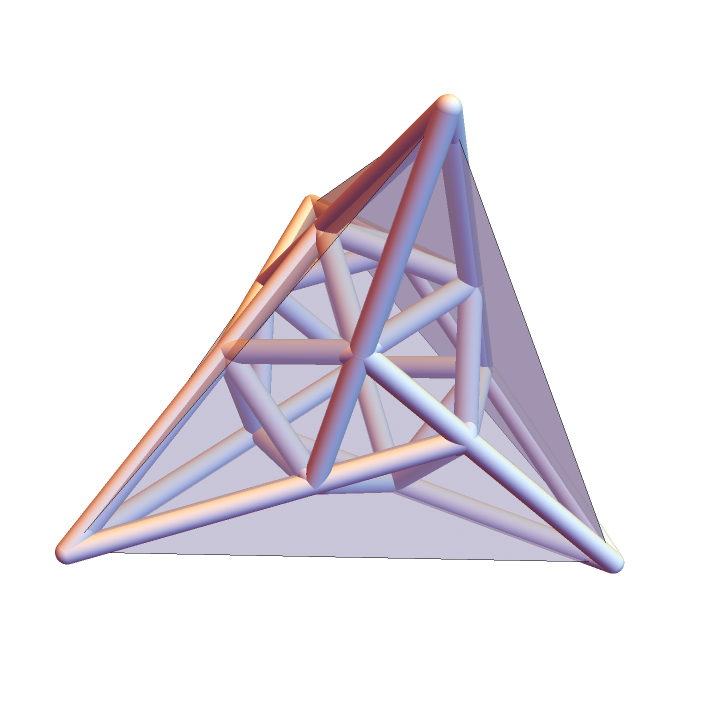## Version History

• 1.0.1 – 26 May 2021